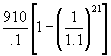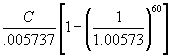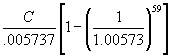Dr. P.V. Viswanath
 Home Bio Courses Research Economics/Finance on the Web Student Interest

 Home/ MBA 632/ Exams/Fall 2003

Quiz 1

1. Please define the following terms briefly:
a. Agency problem
b. Liquidity
c. Free Cash flow
d. Times-interest-earned ratio

2. Please answer the following questions in brief (no more than five sentences):
a. What sorts of firms are more likely to pay out their earnings in dividends?
b. Give two examples of financial institutions and describe briefly what they do.
c. What should be the objective of the manager of a corporation?

a. Myrtle Golf, Inc. has a total debt ratio of 0.55. What is its debt-equity ratio? What is its equity multiplier?
b. Keegan Corporation has ending inventory of \$900,123 and cost of goods sold for the year just ended was \$1, 900,127. What is the inventory turnover? The days’ sales in inventory? How long on average did a unit of inventory sit on the shelf before it was sold?
c. The December 31, 2001 balance sheet of Ann’s Soccer shop showed current assets of \$880 and current liabilities of \$300. The December 31, 2002 balance sheet showed current assets of \$840 and current liabilities of \$402. What was the company’s 2002 change in net working capital or NWC?

Solutions to Quiz 1

1. a. An agency problem arises when there is a conflict of interests in a principal-agency situation, i.e. when somebody (a principal) appoints/hire/relies upon another person (an agent) to work on his behalf. Because of this conflict of interests, suboptimal actions may be taken by the agent. An example of this is the conflict of interest between the owners and management of a firm.
b. liquidity refers to the ease and speed with which an asset can be converted to cash.
c. Free Cash Flow refers to the cash flow generated from assets that is available for distribution to equityholders or other securityholders.
d. Times-interest-earned ratio refers to a measure of solvency and equals EBIT ¸ Interest.

2.a. Firms that have more cashflow generated than they have avenues to reinvest in, profitably, are likely to pay more of their earnings out in dividends.
b. A bank and an insurance company are two examples of financial institutions. A bank takes in demand and term deposits and lends them out to corporations and other businessmen. An insurance company helps individuals pool different kinds of risks, such as death risk or catastrophe risk.
c. From a fiduciary perspective, the objective of a firm’s manager should be to maximize the stock price. From a long run, ex-ante perspective, it should be to maximize the firm’s value and/or the contribution of the firm to society.

3. a. If Myrtle has a debt ratio of 0.55, Debt ¸ Total Assets = 0.55; hence Debt ¸ Equity = 0.55/0.45 or 1.22. The equity multiplier is 2.22 (1+ the debt-equity ratio).
b. Inventory Turnover equals Cost of Goods Sold/Inventory = 1,900,127/900,123 = 2.11. Days Sales in Inventory equals = 365/Inventory Turnover = 172.91 days; this means that, on average, a unit of inventory sat for these many days, i.e. 172.91 on the shelf before it was sold.
c. Net Working Capital for 2002 is 840-402 = 438; NWC for 2001 is 880-300 = 580; hence change in NWC = 438-580 = -\$142.

Quiz 2

1. What is the present value of \$910 per year, at a discount rate of 10 percent, if the first payment is received 5 years from now and the last payment is received 25 years from now?

2. a. If your bank pays you \$103.25 after 6 months, for an initial deposit of \$100, what is the effective annual interest rate that it is paying?

2. b. Your bank has a general policy of lending at an interest rate 0.5% higher than the interest rate that it pays its customers on their deposits. If you wanted to borrow \$250,000 from your bank, and you wanted to repay the loan in monthly instalments, how much would you have to pay the bank every month for 60 months? (Note: the first payment will start at the end of the month.)

Bonus: If you offered to start the payments right away (i.e. at the beginning of the month), what would your monthly payments be?

Solutions to Quiz 2

1. The present value of the payments at the beginning of year 5 (end of year 4) is simply the present value of an annuity:= \$7870.31. Bringing it back to the beginning of year 1, we get 7870.31/(1.1)4 = \$5375.53.

2a. The effective annual interest rate is [(103.25)/100]2-1=0.06606 or 6.606%.

2b. The lending rate is, therefore, 6.606 + .5 or 7.106%.
To get the monthly payment, we must first figure out the monthly effective rate. This is obtained by taking computing (1.07106)(1/12)-1, which equals 0.005737 or 0.5737%.

We now solve the equation: 250000 =, or C = \$4936.65.

Bonus: If you offered to make the payments at the beginning of the month, you would solve the equation: 250,000 = C +, or C = \$4908.49.

Quiz 2 Alternate

1. Mullineaux Co. issued 11-year bonds one year ago at a coupon rate of 7.50 percent. The bonds make semi-annual payments. If the yield to maturity on these bonds is 8.5 percent, what is the current bond price? If the bonds were issued without coupons (i.e. if the coupon rate were set at zero), how much larger would the bond issue have to be (measured in terms of the face value of the bonds sold), in order to raise the same amount of money?

2. You are examining whether your savings will be adequate to meeting your retirement needs. You saved \$150 last month (which was your first month of saving), and you expect your annual savings to grow 1% a month for the next 15 years. If you can invest your money at an effective annual rate of 8%, how much would you expect to have at the end of the fifth year?

Solutions to Quiz 2 Alternate

1. The coupon rate is 7.5%; with semi-annual coupon payments, that makes for a dollar payment of \$37.50. Since the bonds were issued 1 year ago, and they were originally 11 year bonds, they are now 10 year bonds. Discounting the present value of all the coupons at the semi-annual rate of 8.5/2.25%, we get a bond price of \$933.5282 or \$933.53, after rounding.
If the bonds were sold without coupons today, the face value would have to be equal to (933.5282)*(1.0425)20, which yields \$2146.09.

2. We can compute this by first obtaining the present value of a growing annuity, and then computing the future value. We first compute the effective monthly rate, which works out to 1.08(1/12) = 0.6434%. We can now compute the present value as
150 + (150)(1.01)[1-(1.01/1.006434)60]/(0.006434 - 0.01)= \$10,194.00. The future value is 10,194(1.08)5 = \$14978.33

Quiz 3

1. Please define any three of the following terms briefly (5 points each):
a. specialist
b. inside quote
c. preemptive right
d. cumulative voting

2. Please answer two of the following three questions in brief (no more than half a page) (15 points each):
a. Why would somebody buy a stock that does not pay a dividend, and furthermore, has never paid a dividend in the past?
b. Describe how you might go about valuing a stock that has never paid a dividend.
c. Consider the following two ways of pricing a stock: one, to compute the present value of all future dividend payments; two, to compute the present value of the next dividend (one period from now) and the present value of the price at which you expect to be able to sell the stock next period (just after you receive the dividend). Under what circumstances might the two valuations diverge?

 Year A B 0 -38,000 -70000 1 16000 10000 2 19000 15000 3 18000 20000 4 5000 80000

a. Which of the two projects, A and B, will have a higher IRR? (Answer without using a calculator.) Explain. No points without an explanation. ( 10 points)

b. Which of the two projects will have a positive IRR? (5 points)

1. A and B
2. A, but not B
3. B, but not A
4. neither A, nor B

c. Consider the following two projects, and answer the questions below:

 Year A B 0 -300 -300 1 100 80 2 100 90 3 100 100 4 100 110 5 100 140 IRR 19.86% 19.49%
1. If you used the IRR rule, which of the two projects would you accept, assuming you could only accept one of them? (5 points)
2. Compute the present value of the two projects at a discount rate of 5%. If the true discount rate were indeed 5%, which of the two projects would you accept using the NPV rule? (15 points)
3. Your friend says that there is a discount rate less than 19.49% for which you would actually pick project A over project B. Prove him right or wrong. (20 points)

4. In one of your classes, your professor says that decimalization is bad for investors. Explain how he might be right (10 bonus points; no more than one page).

Solutions to Quiz 3

1. a. A specialist is a NYSE member acting as a dealer in a small number of securities on the exchange floor. He is required to ensuring a smooth and orderly price path.
b. inside quote refers to the highest bid and the lowest ask quotes for a security
c. preemptive right refers to the right of existing shareholders to share proportionally in any new stock sold.
d. cumulative voting is a procedure whereby a shareholder may cast all votes for one member of hte board of directors. It allows minority shareholders to obtain representation on the Board.

2. a. Even if the stock has never paid a dividend before, a shareholder might want to buy it for future dividends. It could be that dividends were not been paid in the past because of reinvestment of earnings.
b. We'd have to estimate future dividends, both the quantity and when they would be paid. We could then discount them to the present as with any cashflow model, in order to get the stock price.
c. The two approaches would diverge if we thought that the stock was not being priced correctly. If we believed that the stock would be overpriced next year (or whenever we planned to sell it), our true value would be the discounted present value of next year's dividend and selling price.

3. a. In this case, both A and B have an IRR = 0 because the sum of all the cash inflows equals the initial investment. Normally, one might have said that Project A would have a higher IRR because the total cashflow for both projects is the same, while those cashflows occur sooner with project A.
b. Neither A nor B will have a positive IRR because the sum of the cashflows is equal to the initial investment. In fact, the IRR will be zero for both A and B.
c. i) Project A would be accepted because it has a higher IRR.
ii) The NPV works out to \$132.95 for project A and \$144.40 for project B.
iii) Your friend is right. This is because the IRR for project B is 19.49%, but 19.86 for A; this means that at a discount rate of 19.49%, the NPV for project A is > 0, while it is zero for B. That is, NPV(A) > NPV(B). However, at 5%, NPV (B) > NPV (A). Hence, as we move from a discount rate of 5% to 19.49%, the NPVs of the two projects must be equal. This means that for some discount rate lower than 19.49%, NPV(A) must still be higher than NPV(B).

4. Decimalization could reduce liquidity. This is because market makers will have less of an incentive to place limit orders. When a market maker provides a quote for a stock, he is saying that I will stand ready to buy (or sell) a minimum number of shares at that price. If anybody wants to take away a potential sale/purchase (and the associated profits) from the market maker, they would have to improve on the price offered by the market maker. With decimalization, other traders could do this for the cost of a single cent per share. Hence the expected profit for the market maker from making a market (i.e. putting out a quote) is less; this means that he is less likely to do so under decimalization. This makes for higher bid-ask spreads, which means worse prices if an investor wants to buy or sell with a market order.